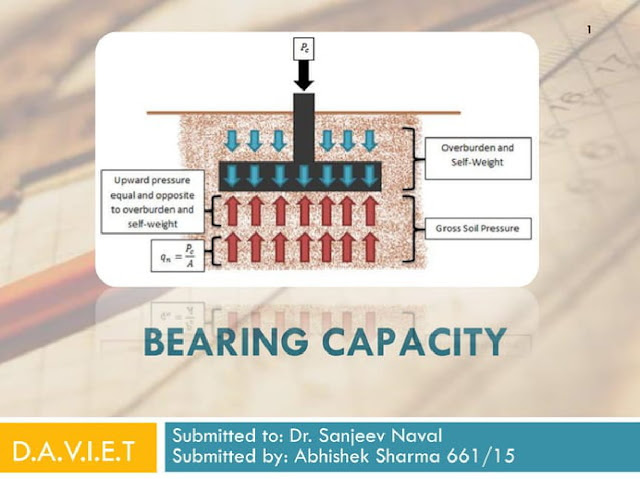## Tuesday, January 3, 2023

### Introduction on Bearing Capacity - PDFWhat is the bearing capacity of soil?
In a nutshell, bearing capacity is the capacity of soil to support the loads that are applied to the ground above. It depends primarily on the type of soil, its shear strength and its density. It also depends on the depth of embedment of the load – the deeper it is founded, the greater the bearing capacity.

Where there is insufficient bearing capacity, the ground can be improved or alternatively the load can be spread over a larger area such that the applied stress to the soil is reduced to an acceptable value less than the bearing capacity. This can be achieved with spread foundations composed of reinforced concrete, for example.

In the case of working platforms for cranes and piling rigs, improved load spread is provided by a granular platform whose performance can be further improved by mechanical  stabilisation using Tensar geogrids.

Bearing capacity types and formulae

The types of bearing capacity of soil are:

• Ultimate bearing capacity (qᵤ): the maximum vertical pressure that can be applied to the ground surface, at which point a shear failure mechanism develops in the supporting soil.
• Net ultimate bearing capacity (qᵤ): this is the ultimate bearing capacity minus the weight of soil (𝝲) multiplied by the depth of the foundation (D). The formula is qₙᵤ = qᵤ - 𝝲Df.
• Net safe bearing capacity (qₙₛ): the allowable bearing capacity  (qₙₛ) is the net ultimate bearing capacity (qᵤ) divided by a factor a safety (typically this will be 3). The formula is qₙₛ = qᵤ / F. The factor may be increased to limit settlements further if required.
• Gross safe bearing capacity (qₛ): dividing the ultimate bearing capacity by a factor of safety gives you the gross safe bearing capacity (qₛ = qᵤ / F).
• Net safe settlement pressure (qₙₚ): the maximum load the soil can take before it exceeds the allowable amount of soil settlement.‎
• Net allowable bearing capacity (qₙ‎ₐ): this is the value used in the design of foundations, and is often simply referred to as the ‘allowable bearing capacity’. The net allowable bearing capacity (qₙ‎ₐ) is equal to either the net safe bearing capacity (qₙₛ) or the net safe settlement pressure (qₙₚ), whichever is the lower figure.